# C++函数及其应用

函数是编程很重要的一部分，他能给程序带来很多益处，也方便我们程序员编写代码。

1. 我们知道，c和c++中使用函数，能简化代码量，对各个部分进行封装，使得问题变得简单和直观，提高了程序的易读性。

2. 还可以提升可维护性，把一些计算或操作编成通用的函数，以供随时调用，从而避免了代码的重复冗长。

3. 但是运用函数，就需要传递参数，开辟缓存、堆栈等，相比较而言，会耗一些多余的效率。

#include <iostream>
using namespace std;
void func(int a,int b,int c)
{
int middle,max,small;
if(a>b){
max=a;
middle =b;
}
else{
max=b;
middle =a;
}

if(c>max){
cout << c << " " << max << " "<< middle;
}
else if(c<middle){
cout << max << " " << middle << " " <<c;
}
else{
cout << max << " " << c << " " <<middle;
}
}

int main(){
int a,b,c;
cout << "请输入a,b,c的大小\n";
cin >> a >> b >> c;

func(a,b,c);//进行排序

return 0;
}


我们可以看出用一个func函数对三个数进行比较大小后，主函数就变得简单清晰，程序可读性变强。

在C++中，如果需要定义几个功能相似，而参数类型不同的函数，那么这样的几个函数可以使用相同的函数名，这就是“函数重载”。

1、函数的参数个数不同；

2、函数的参数类型不同或者参数类型顺序不同。

#include<iostream>
using namespace std;

int sum(int a=0,int b=0){
return a+b;
}
double sum(double a=0,double b=0){
return a+b;
}
float sum(float a=0,float b=0,float c=0){
return a+b+c;
}

int main(){

int x=sum(5,9);
float y=sum(2.7,5.87);
float z=sum(float(x),y,5);

cout << x << " " << y << " " << z;
return 0;
}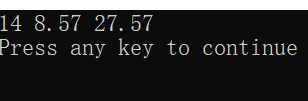调用时，将实参的值传递对应的形参，即为值传递。函数中对任何形参值得修改都不会改变实参变量的值。

#include<iostream>
using namespace std;

void swap(int x,int y){

int temp;

temp=x;
x=y;
y=temp;

cout << "swap中a和b " << x << " " << y << endl;

}

int main(){

int a,b;

cin >> a >> b;

swap(a,b);

cout << "main中a和b " << a << " " << b << endl;

return 0;
}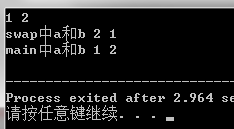我们分析一下整个程序：在主函数中，实参a和b有自己的存储空间，并且有自己的初始值。当调用函数Swap时，内存给函数的参数x和y分配存储空间，并将a和b的值复制过来，函数执行过程中，将x和y的值进行交换，当函数执行结束之后，x和y所占用的存储空间将被释放，这种传递的方式，并不会对实参a和b的值产生影响，所以看到的a和b的值没哟改变。

引用变量是变量的另一个别名，它没有自己的存储数据的内存位置，它访问的是另一个变量的内存位置。对引用变量作出的任何更改，实际上都是对它所引用的变量内存位置中存储数据的更改。

#include<iostream>
using namespace std;

void swap(int &x,int &y){

int temp;

temp=x;
x=y;
y=temp;

cout << "swap中a和b " << x << " " << y << endl;

}

int main(){

int a,b;

cin >> a >> b;

swap(a,b);

cout << "main中a和b " << a << " " << b << endl;

return 0;
}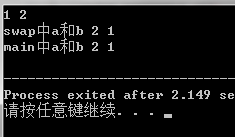说起递归，不得不说他很想小时候听过的故事：从前有座山，山里有座庙，庙里有个和尚，和尚在讲故事，从前有座山，山里有座庙，庙里有个和尚，和尚在讲故事，从前有座山...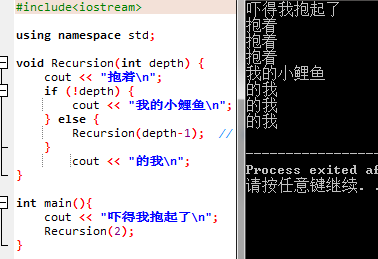我们再来看看不好理解的汉罗塔问题：该问题是在一块铜板装置上，有三根杆(编号A、B、C)，在A杆自下而上、由大到小按顺序放置n个金盘。游戏的目标：把A杆上的金盘全部移到C杆上，并仍保持原有顺序叠好。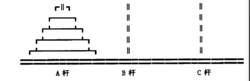研究这个问题我们就要利用递归思想，要把n个盘子从A->C，我们就要先把n-1个盘子从A->C->B，再把A最后一个盘子从A->C，紧接着再把n-1个盘子从B->A->C上。

//栈之递归汉诺塔问题
#include<iostream>

using namespace std;

void move(int n,char x,char y,char z){

if(n==1)
cout << x <<"->" << z << endl;  //把x放到z上

else{

move(n-1,x,z,y);
cout << x <<"->" << z << endl;
move(n-1,y,x,z);

}
}

int main(){

move(3,'x','y','z');

return 0;

}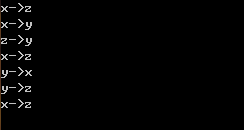posted on 2019-09-14 23:31  夏千意  阅读(2790)  评论(0编辑  收藏  举报